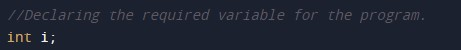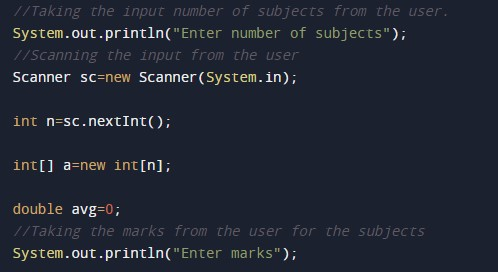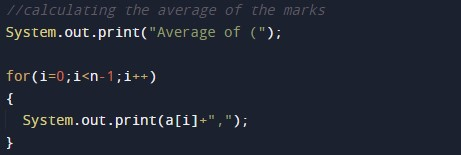# Java Program to calculate Marks Average

In this tutorial you will learn about the Java Program to calculate Marks Average and its application with practical example.

## Java Program to calculate Marks Average

In this tutorial, we will learn to create a Java program that will calculate Marks Average using Java programming.

## Prerequisites

Before starting with this tutorial, we assume that you are the best aware of the following Java programming topics:

• Operators in Java Programming.
• Basic Input and Output function in Java Programming.
• Basic Java programming.
• Arithmetic operations in Java Programming.

## What is a percentage of numbers?

The percentage of all the marks is calculated by adding the total marks. And then we will divide it by the maximum marks and multiplied by 100.

## What is the average of numbers?

The average of numbers in mathematics means that all the numbers are added and then the sum of all the numbers is divided by the total number of objects.

It means if there are 5 subject marks as follows {60,60,60,80,70} then the average will be:-

average = 60+60+60+80+70/5

average = 60.

## Program to calculate Average of marks.

In this program, we will first take the marks of all five subjects from the user. And then we will add them to find the total marks. Now the total will be divided by the number of subjects to find the average.  Then the total will divide by maximum marks by 5. Last, we will print the output average marks.

Example using the below program.

## Output:-In the above program, we have first initialized the required variable.• i = it will hold the integer value.
• average = it will hold the float value.
• a = it will hold the integer value.

Input number of subjects and the marks from the user.Program Logic Code.Printing output.In this tutorial we have learn about the Java Program to calculate Marks Average and its application with practical example. I hope you will like this tutorial.